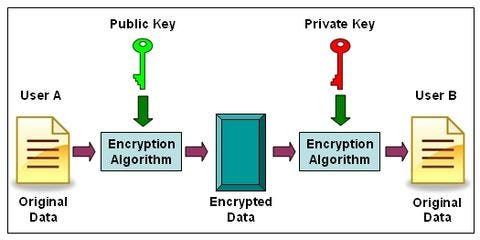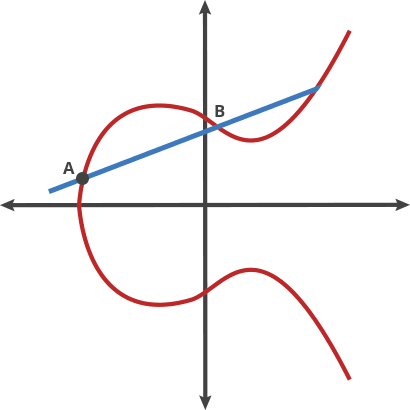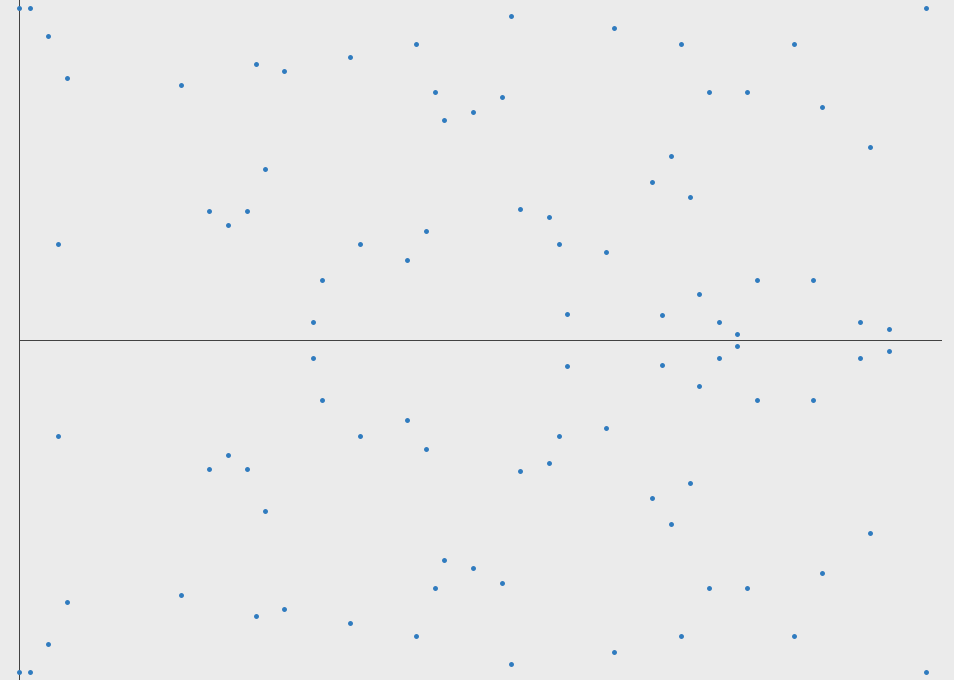# (Very) Basic Intro To Elliptic Curve Cryptography

The post (Very) Basic Intro To Elliptic Curve Cryptography appeared first on Qvault.

This is going to be a basic introduction to elliptic curve cryptography. I will assume most of my audience is here to gain an understanding of why ECC is an effective cryptographic tool and the basics of why it works. My goal is to explain it in a general sense, I will be omitting proofs and implementation details and instead focusing on the high-level principles of what makes it work.

### What It’s For?

ECC is a way to encrypt data so that only specific people can decrypt it. This has several obvious real-life use cases, but the main usage is in encrypting internet data and traffic. For instance, ECC can be used to ensure that when an email is sent, no one but the recipient can read the message.

### ECC is a type of Public Key Cryptography

There are many types of public-key cryptography, and Elliptic Curve Cryptography is just one flavor. Other algorithms include RSA, Diffie-Helman, etc. I’m going to give a very simple background of public-key cryptography in general as a starting point so we can discuss ECC and build on top of these ideas. Please, by all means, study more in-depth on public-key cryptography when you have the time.

As seen below, public-key cryptography allows the following to happen:The graphic shows two keys, a public key, and a private key. These keys are used to encrypt and decrypt data so that anyone in the world can look at the encrypted data while it is being transmitted, and be unable to read the message.

Let’s pretend that Facebook is going to receive a private post from Donald Trump. Facebook needs to be able to ensure that when the President sends his post over the internet, no one in the middle (Like the NSA, or internet service provider) can read the message. The entire exchange using Public Key Cryptography would go like this:

• Donald Trump Notifies Facebook that he wants to send them a private post
• Facebook sends Donald Trump their public key
• Donald Trump uses the public key to encrypt his post:

“I love Fox and Friends” + Public Key = “s80s1s9sadjds9s”

• Donald Trump sends only the encrypted message to Facebook
• Facebook uses its private key to decrypt the message:

“s80s1s9sadjds9s” + Private Key= “I love Fox and Friends”

As you can see this is a very useful technology. Here are some key points.

• The public key can be sent to anyone. It is public.
• The private key must be kept safe because if someone in the middle were to get the private key they could decrypt the messages.
• Computers can very quickly use the public key to encrypt a message, and the private key to decrypt a message.
• Computers require a very long time (millions of years) to derive the original data from the encrypted message if they don’t have the private key.

### How it Works: The Trapdoor Function

The crux of all public-key cryptographic algorithms is that they each have their own unique trapdoor function . A trapdoor function is a function that can only be computed one way, or at least can only be computed one way easily (in less than millions of years using modern computers).

Not a trapdoor function: A + B = C

If I’m given A and B I can compute C. The problem is that if I’m given B and C I can also compute A. This is not a trapdoor function.

Trapdoor function:

“I love Fox and Friends” + Public Key = “s80s1s9sadjds9s”

If given “I love Fox and Friends” and the public key, I can produce “s80s1s9sadjds9s”, but if given “s80s1s9sadjds9s” and the Public Key I can’t produce “I love Fox and Friends”

In RSA (Probably the most popular public-key system) the trapdoor function relies on how hard it is to factor large numbers into their prime factors.

Public Key: 944,871,836,856,449,473

Private Key: 961,748,941 and 982,451,653

In the example above the public key is a very large number, and the private key is the two prime factors of the public key. This is a good example of a Trapdoor Function because it is very easy to multiply the numbers in the private key together to get the public key, but if all you have is the public key it will take a very long time using a computer to re-create the private key.

Note: In real cryptography, the private key would need to be 200+ digits long to be considered secure.

### What Makes Elliptic Curve Cryptography Different?

ECC is used for the exact same reasons as RSA. It simply generates a public and private key and allows two parties to communicate securely. There is one major advantage however that ECC offers over RSA. A 256 bit key in ECC offers about the same security as 3072-bit key using RSA. This means that in systems with limited resources such as smartphones, embedded computers, cryptocurrency networks, it uses less than 10% of the hard disk space and bandwidth required using RSA.

### ECC’s Trapdoor Function

This is probably why most of you are here. This is what makes ECC special and different from RSA. The trapdoor function is similar to a mathematical game of pool. We start with a certain point on the curve. We use a function (called the dot function) to find a new point. We keep repeating the dot function to hop around the curve until we finally end up at our last point. Lets walk through the algorithm.• Starting at A:
• A dot B = -C (Draw a line from A to B and it intersects at -C)
• Reflect across the X axis from -C to C
• A dot C = -D (Draw a line from A to C and it intersects -D)
• Reflect across the X axis from -D to D
• A dot D = -E (Draw a line from A to D and it intersects -E)
• Reflect across the X axis from -E to E

This is a great trapdoor function because if you know where the starting point (A) is and how many hops are required to get to the ending point (E), it is very easy to find the ending point. On the other hand, if all you know is where the starting point and ending point are, it is nearly impossible to find how many hops it took to get there.

Public Key: Starting Point A, Ending Point E

Private Key: Number of hops from A to E

### Questions?

Here are just a couple of questions I had when I first learned about ECC. Hopefully, I can address them properly.

### How is the second point found? If the dot function is basically drawing a line between two points, don’t you need a second point to start with?

Answer: No. The second point (we will call it -R below) is actually the result of P dot P (let’s assume the first point is called P)

P dot P= -R

So what is P dot P? It is actually just the tangent line of P. See the graphic below:### What happens if the dot function produces a line that will go way off out to some extreme?

If the line doesn’t hit the curve close to the origin, we can actually define a maximum X value where the line will wrap back around and start from the beginning again. See the graphic below for an example.### I get the trapdoor function, but how are public and private keys created in practice? How are they used in conjunction with data to be encrypted?

This is a great question, but it requires a much more in-depth answer. I gave very hand-wavy explanations of both RSA and ECC in this article. However, there are plenty of more technical resources out there and I encourage you to look into them.

### Who Cares?

I first studied ECC because of my interest in Bitcoin and cryptocurrencies. ECC is used as the cryptographic key algorithm cryptocurrencies today because it uses less than 10% of the key size (and by extension data) of RSA.

I hope you learned a bit about ECC and public-key cryptography, this is by no means a technical description. I hope to give more people an interest in the capabilities of ECC and general knowledge of why it works.